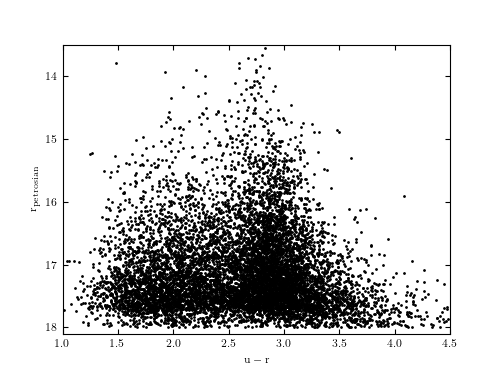# SDSS Spectroscopic Galaxy Sample¶

Figure 1.3.

The r vs. u-r color-magnitude diagram for the first 10,000 entries in the catalog of spectroscopically observed galaxies from the Sloan Digital Sky Survey (SDSS). Note two “clouds” of points with different morphologies separated by u-r ~ 2.3. The abrupt decrease of the point density for r > 17.7 (the bottom of the diagram) is due to the selection function for the spectroscopic galaxy sample from SDSS. This example shows how to fetch photometric data from the SDSS spectroscopic sample and plot a simple color-magnitude diagram.# Author: Jake VanderPlas
#   The figure produced by this code is published in the textbook
#   "Statistics, Data Mining, and Machine Learning in Astronomy" (2013)
#   To report a bug or issue, use the following forum:
import numpy as np
from matplotlib import pyplot as plt
from astroML.datasets import fetch_sdss_specgals

#----------------------------------------------------------------------
# This function adjusts matplotlib settings for a uniform feel in the textbook.
# Note that with usetex=True, fonts are rendered with LaTeX.  This may
# result in an error if LaTeX is not installed on your system.  In that case,
# you can set usetex to False.
if "setup_text_plots" not in globals():
from astroML.plotting import setup_text_plots
setup_text_plots(fontsize=8, usetex=True)

#------------------------------------------------------------
# Fetch spectroscopic galaxy data
data = fetch_sdss_specgals()
data = data[:10000]

u = data['modelMag_u']
r = data['modelMag_r']
rPetro = data['petroMag_r']

#------------------------------------------------------------
# Plot the galaxy colors and magnitudes
fig, ax = plt.subplots(figsize=(5, 3.75))
ax.plot(u - r, rPetro, '.k', markersize=2)

ax.set_xlim(1, 4.5)
ax.set_ylim(18.1, 13.5)

ax.set_xlabel(r'$\mathrm{u - r}$')
ax.set_ylabel(r'$\mathrm{r_{petrosian}}$')

plt.show()